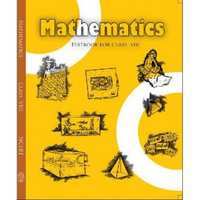# NCERT solutions for Class 8 Maths chapter 12 - Exponents and Powers [Latest edition]

#### Chapters## Chapter 12: Exponents and Powers

Exercise 12.1Exercise 12.2
Exercise 12.1 [Pages 197 - 198]

### NCERT solutions for Class 8 Maths Chapter 12 Exponents and PowersExercise 12.1 [Pages 197 - 198]

Exercise 12.1 | Q 1.1 | Page 197

Evaluate 3−2

Exercise 12.1 | Q 1.2 | Page 197

Evaluate (−4)−2

Exercise 12.1 | Q 1.3 | Page 197

Evaluate (1/2)^(-5)

Exercise 12.1 | Q 2.1 | Page 197

Simplify and express the result in power notation with positive exponent.

(-4)5 ÷ (-4)8

Exercise 12.1 | Q 2.2 | Page 197

Simplify and express the result in power notation with positive exponent.

(1/2^3)^2

Exercise 12.1 | Q 3.1 | Page 197

Find the value of.
(30 + 4−1) × 22

Exercise 12.1 | Q 3.2 | Page 197

Find the value of.
(2−1 × 4−1) ÷2−2

Exercise 12.1 | Q 3.3 | Page 197

Find the value of.

(1/2)^(-2)+(1/3)^(-2)+(1/4)^(-2)

Exercise 12.1 | Q 3.4 | Page 197

Find the value of (3−1 + 4−1 + 5−1)0

Exercise 12.1 | Q 3.5 | Page 197

Find the value of {((-2)/3)^(-2)}^2

Exercise 12.1 | Q 4.1 | Page 198

Evaluate (8^(-1)xx5^(3))/2^(-4)

Exercise 12.1 | Q 4.2 | Page 198

Evaluate (5^(-1)xx2^(-1))xx6^(-1)

Exercise 12.1 | Q 5 | Page 198

Find the value of m for which 5m ÷5−3 = 55.

Exercise 12.1 | Q 6.1 | Page 198

Evaluate  {(1/3)^(-1) - (1/4)^(-1)}^(-1)

Exercise 12.1 | Q 6.2 | Page 198

Evaluate (5/8)^(-7) xx (8/5)^(-4)

Exercise 12.1 | Q 7.1 | Page 198

Simplify (25 xx t^(-4))/(5^(-3) xx10xxt^(-5)) (t != 0)

Exercise 12.1 | Q 7.2 | Page 198

Simplify (3^(-5) xx 10^(-5) xx 125)/(5^(-7) xx 6^(-5))

Exercise 12.2 [Page 200]

### NCERT solutions for Class 8 Maths Chapter 12 Exponents and PowersExercise 12.2 [Page 200]

Exercise 12.2 | Q 1.1 | Page 200

Express the given numbers in standard form.

0.0000000000085

Exercise 12.2 | Q 1.2 | Page 200

Express the given numbers in standard form.

0.00000000000942

Exercise 12.2 | Q 1.3 | Page 200

Express the given numbers in standard form.

6020000000000000

Exercise 12.2 | Q 1.4 | Page 200

Express the given numbers in standard form.

0.00000000837

Exercise 12.2 | Q 1.5 | Page 200

Express the given numbers in standard form.

31860000000

Exercise 12.2 | Q 2.1 | Page 200

Express the given numbers in usual form.

3.02 × 10−6

Exercise 12.2 | Q 2.2 | Page 200

Express the given numbers in usual form.

4.5 × 104

Exercise 12.2 | Q 2.3 | Page 200

Express the given numbers in usual form

3 × 10−8

Exercise 12.2 | Q 2.4 | Page 200

Express the given numbers in standard form.

1.0001 × 109

Exercise 12.2 | Q 2.5 | Page 200

Express the given numbers in standard form.

5.8 × 1012

Exercise 12.2 | Q 2.6 | Page 200

Express the given numbers in standard form.

3.61492 × 106

Exercise 12.2 | Q 3.1 | Page 200

Express the number appearing in the given statements in standard form

1 micron is equal to 1/1000000 m

Exercise 12.2 | Q 3.2 | Page 200

Express the number appearing in the given statements in standard form

Charge of an electron is 0.000, 000, 000, 000, 000, 000, 16 coulomb.

Exercise 12.2 | Q 3.3 | Page 200

Express the number appearing in the given statements in standard form

Size of a bacteria is 0.0000005 m

Exercise 12.2 | Q 3.4 | Page 200

Express the number appearing in the given statements in standard form

Size of a plant cell is 0.00001275 m

Exercise 12.2 | Q 3.5 | Page 200

Express the number appearing in the given statements in standard form

Thickness of a thick paper is 0.07 mm

Exercise 12.2 | Q 4 | Page 200

In a stack there are 5 books each of thickness 20 mm and 5 paper sheets each of thickness 0.016 mm. What is the total thickness of the stack?

## Chapter 12: Exponents and Powers

Exercise 12.1Exercise 12.2## NCERT solutions for Class 8 Maths chapter 12 - Exponents and Powers

NCERT solutions for Class 8 Maths chapter 12 (Exponents and Powers) include all questions with solution and detail explanation. This will clear students doubts about any question and improve application skills while preparing for board exams. The detailed, step-by-step solutions will help you understand the concepts better and clear your confusions, if any. Shaalaa.com has the CBSE Class 8 Maths solutions in a manner that help students grasp basic concepts better and faster.

Further, we at Shaalaa.com provide such solutions so that students can prepare for written exams. NCERT textbook solutions can be a core help for self-study and acts as a perfect self-help guidance for students.

Concepts covered in Class 8 Maths chapter 12 Exponents and Powers are Powers with Negative Exponents, Use of Exponents to Express Small Numbers in Standard Form, Comparing Very Large and Very Small Numbers, Concept of Exponents, Negative Exponents and Laws of Exponents, Decimal Number System Using Exponents and Powers.

Using NCERT Class 8 solutions Exponents and Powers exercise by students are an easy way to prepare for the exams, as they involve solutions arranged chapter-wise also page wise. The questions involved in NCERT Solutions are important questions that can be asked in the final exam. Maximum students of CBSE Class 8 prefer NCERT Textbook Solutions to score more in exam.

Get the free view of chapter 12 Exponents and Powers Class 8 extra questions for Class 8 Maths and can use Shaalaa.com to keep it handy for your exam preparation